## An investigator compares the durability of two different compounds used in the manufacture of a certain automobile brake lining. A sample of

Question

An investigator compares the durability of two different compounds used in the manufacture of a certain automobile brake lining. A sample of 243 brakes using Compound 1 yields an average brake life of 37,866 miles. A sample of 268 brakes using Compound 2 yields an average brake life of 45,789 miles. Assume that the population standard deviation for Compound 1 is 4414 miles, while the population standard deviation for Compound 2 is 2368 miles. Determine the 95% confidence interval for the true difference between average lifetimes for brakes using Compound 1 and brakes using Compound 2. Step 2 of 3 : Calculate the margin of error of a confidence interval for the difference between the two population means. Round your answer to six decimal places.

in progress 0
6 months 2021-08-28T11:03:49+00:00 1 Answers 1 views 0

The margin of error is of 623.2016.

The 95% confidence interval for the true difference between average lifetimes for brakes using Compound 1 and brakes using Compound 2 is (-8546.2016, -7299.7984).

Step-by-step explanation:

Before building the confidence interval, we need to understand the central limit theorem, and subtraction between normal variables.

Central Limit Theorem

The Central Limit Theorem estabilishes that, for a normally distributed random variable X, with mean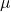and standard deviation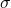, the sampling distribution of the sample means with size n can be approximated to a normal distribution with meanand standard deviation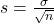.

For a skewed variable, the Central Limit Theorem can also be applied, as long as n is at least 30.

Subtraction between normal variables:

When two normal variables are subtracted, the mean is the subtraction of the means while the standard deviation is the square root of the sum of the variances.

A sample of 243 brakes using Compound 1 yields an average brake life of 37,866 miles. The population standard deviation for Compound 1 is 4414 miles.

This means that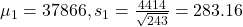A sample of 268 brakes using Compound 2 yields an average brake life of 45,789 miles. The population standard deviation for Compound 2 is 2368 miles.

This means that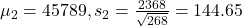Distribution of the difference between average lifetimes for brakes using Compound 1 and brakes using Compound 2.

The mean is: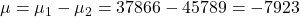The standard deviation is: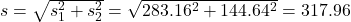Confidence interval:

We have that to find our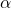level, that is the subtraction of 1 by the confidence interval divided by 2. So: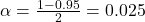Now, we have to find z in the Ztable as such z has a pvalue of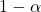.

That is z with a pvalue of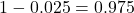, so Z = 1.96.

Now, find the margin of error M as such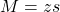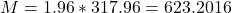The margin of error is of 623.2016.

The lower end of the interval is the sample mean subtracted by M. So it is -7923 – 623.2016 = -8546.2016

The upper end of the interval is the sample mean added to M. So it is -7923 + 623.2016 = -7299.7984

The 95% confidence interval for the true difference between average lifetimes for brakes using Compound 1 and brakes using Compound 2 is (-8546.2016, -7299.7984).